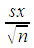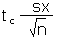# MS 150 Statistics t3 • Name:

LocationSound speed (ms⁻¹)
B bldg diagonal200
Bookstore 270
South faculty 275
West library 286
B bldg diagonal287
Girl's dorm 296
East wall gym 312
Cafeteria 320
West library 326
East wall gym 336
North wall gym 353
North wall gym 390

The data is based on data from a physical science experiment that investigated the speed of sound. 1, 2

1. __________ Find the sample size n for the data.
2. __________ Find the minimum.
3. __________ Find the maximum.
4. __________ Find the range.
5. __________ Find the midrange.
6. __________ Find the median.
7. __________ Find the mode.
8. __________ Find the sample mean x.
9. __________ Find the sample standard deviation sx.
10. __________ Find the sample coefficient of variation CV.
11. __________ If this data is divided into five classes (bins, intervals), what is the width of a single class?
12. Determine the frequency and calculate the relative frequency using five classes. Record your results in the table provided.
Class upper limitsFrequency FRelative Frequency
Sums:
1. Sketch a frequency histogram of the data, labeling your horizontal axis and vertical axis as appropriate.
2. ____________________ What is the shape of the histogram?
3. __________ Theory predicts a speed of sound of 350 ms⁻¹. Calculate the z-score for a sound speed of 350 ms⁻¹ using the mean and standard deviation calculated above.
4. ____________________ Is a z-score for 350 ms⁻¹ ordinary or unusual?
5. ____________________ What is the point estimate for the population mean μ speed of sound?
6. ____________________ Calculate the standard error SE of the mean.
7. ____________________ Calculate the degrees of freedom n − 1.
8. ____________________ Calculate t-critical tc for confidence levels of 95%.
9. ____________________ Calculate the margin of error E for the mean.
10. Calculate the 95% confidence interval for the population mean μ speed of sound.

p(__________ ≤ μ ≤ __________) = 0.95
11. ____________________ Does the 95% confidence interval include 350 ms⁻¹?
12. ____________________ Sound speed theory states that the speed of sound is 350 ms⁻¹. Is 350 ms⁻¹ a possible population mean μ for this sample at a confidence level of 95%?
13. ____________________ Based on the data provided, would we disconfirm or fail to disconfirm a theory that stated the speed of sound is 350 ms⁻¹?
Confidence interval statistics
Statistic or ParameterSymbolEquationsOpenOffice
Degrees of freedomdfn − 1 =COUNT(data)-1
Find a tcritical value from a confidence level c tc =TINV(1-c;df)
Standard error of the sample mean SE=STDEV(data)/SQRT(sample size n)
Calculate a margin of error for the mean E for n ≥ 5 using sx. E=tc*sx/SQRT(n)
Calculate a confidence interval for a population mean μ from a sample mean x and an error tolerance E x − E ≤ μ ≤ x + E